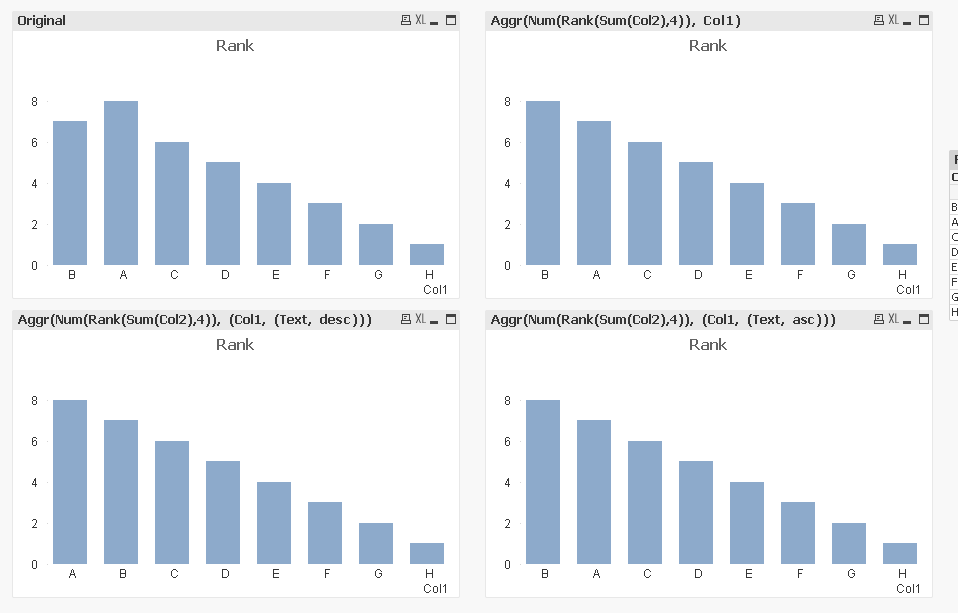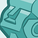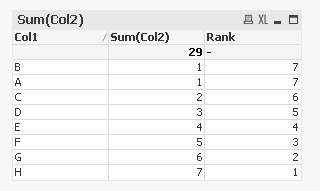# QlikView App Dev

Discussion Board for collaboration related to QlikView App Development.

Announcements
Customer & Partners, DEC. 9, 11 AM ET: Qlik Product & Strategy Roadmap Session: Data Analytics REGISTER NOW
cancel
Showing results for
Did you mean:Partner

## Rank on Sort by Expression

Hi All,

I have two columns, one having dimension and other having value and I m trying create a bar chart and sort the dimension value by Rank function. I m aware that I can sort by y-expression but in my application I pass values in between range and display only those dimensions alone.

The Rank sort works fine but when there is same value in measure, then Rank function doesn't sort correctly even after using Num(Rank(),4). Attached is an sample file of the problem statement.

Labels (1)
• ### Rank Sort by Expression

1 Solution

Accepted SolutionsMVP

I don't really understand the behavior, but what I found is that if I use Aggr() function it fixes the issue.

1. Use Aggr(Num(Rank(Sum(Col2),4)), Col1) for both expression and sorting expression
2. Use Aggr(Num(Rank(Sum(Col2),4)), (Col1, (Text, desc))) for both expression and sorting expression
3. Use Aggr(Num(Rank(Sum(Col2),4)), (Col1, (Text, asc))) for both expression and sorting expression

What confuses me is why do I need the same as expression? If I use Num(Rank(Sum(Col2),4)) as expression and one of the three as sorting expression, it doesn't really work. Also, if I use Num(Rank(Sum(Col2),4)) as sorting expression and one of the three as sorting expression, again it doesn't work.9 RepliesCreator II

This is an interesting question, seems from chart part, only sort by Y axis is the solution. Thanks!Specialist II

maybe this

``Num(Rank(Sum(Col2),1,4))``Partner
Author

My concern is understanding how Rank() function works on Sort by Expression. In the original attachment of QVW file. If you sort the chart by Y-Expression you get a different sorting order. And when you sort the chart by Expression and copy paste the same Expression on Y-Axis to Sort by Expression I get different visual effect.  Shouldn't both the view display same result?MVP

It's a nice exception find I would say; I never noticed this. However, I guess I can explain this behavior. Though I am exactly sure how the QIX is designed. I will try to explain what it seems to me.

Load order plays an important role in sorting while there is a tie. Most probably, when you are sorting by Y-axis, it's 'load order'  sort of dimension values in case of a tie (actually your chart calculation is also based on this logic). However, if you use sort by-Expression, then your sort order defined in expression setting becomes the decider of ranking of values which are in tie.

I am not sure if I could explain it right or not. Hope this helps.

I am also tagging experts for their opinion.

ThanksPartner
Author

Thank you for looking into this post.

Version - 12.10.20600.0 SR8

Sort by expression is not sorting the bars by Rank, as you can see in the below image where lesser Rank of B is sorted first and then followed by A which has higher Rank.Thank you,

Rakul.MVP

I don't really understand the behavior, but what I found is that if I use Aggr() function it fixes the issue.

1. Use Aggr(Num(Rank(Sum(Col2),4)), Col1) for both expression and sorting expression
2. Use Aggr(Num(Rank(Sum(Col2),4)), (Col1, (Text, desc))) for both expression and sorting expression
3. Use Aggr(Num(Rank(Sum(Col2),4)), (Col1, (Text, asc))) for both expression and sorting expression

What confuses me is why do I need the same as expression? If I use Num(Rank(Sum(Col2),4)) as expression and one of the three as sorting expression, it doesn't really work. Also, if I use Num(Rank(Sum(Col2),4)) as sorting expression and one of the three as sorting expression, again it doesn't work.Creator II

Hi Can you try to explain this? Seems the second parameter is to:

mode

The second argument, mode, can take the following values:

Value Description
 0 (default) If all ranks within the sharing group fall on the low side of the middle value of the entire ranking, all rows get the lowest rank within the sharing group.If all ranks within the sharing group fall on the high side of the middle value of the entire ranking, all rows get the highest rank within the sharing group.If ranks within the sharing group span over the middle value of the entire ranking, all rows get the value corresponding to the average of the top and bottom ranking in the entire column segment. 1 Lowest rank on all rows. 2 Average rank on all rows. 3 Highest rank on all rows. 4 Lowest rank on first row, then incremented by one for each row.MVP & Luminary

Without diving deeper in this case I'm not sure if rank() is really a valid sort-option. It's not tested but I could imagine that it behaved a bit similar to rowno() which disabled the sorting at least in the table-charts (maybe the other, too). Further I think it's not really needed because an expression like sum(value) won't create a different result as rank(sum(value)) in regard to a sorting.

- MarcusPartner
Author

HI,

Seems like Aggr() over the Rank along with Text sort works fine. As you said, I m still not able to understand how Agg() function makes a difference when we already have the dimension in dimension list.

Glad that you helped to find a work around for this problem.

Thank you,

Rakul.Community Browser